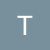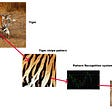# OC: Original Cipher (Shift Cipher aka Caesar Cipher)

`const quotient = (dividend,divisor) =>     Math.floor(dividend/divisor)const floor_modulo = (dividend,divisor) =>     dividend — divisor * quotient(dividend,divisor)`
`const applyAlgoToString = algo => pipe([    map(algo),    join('')])const encryptCharacter = curry((alphabetMap, k, plainCharacter) => {    const alphabetLength = numberOfKeys(alphabetMap)    const x = alphabetMap[plainCharacter]    const y = floor_modulo(x + k, alphabetLength)    const cipherCharacter = findKeyByValue(alphabetMap, y)    return cipherCharacter})const decryptCharacter = curry((alphabetMap, k, cipherCharacter) => {    const alphabetLength = numberOfKeys(alphabetMap)    const y = alphabetMap[cipherCharacter]    const x = floor_modulo(y - k, alphabetLength)    const plainCharacter = findKeyByValue(alphabetMap, x)    return plainCharacter})const shiftCipher = (alphabet, k) => {    const alphabetArray = alphabet.split('')    const alphabetMap = arrayToAlphabetMap(alphabetArray)    const encryptString =           applyAlgoToString(encryptCharacter(alphabetMap, k))    const decryptString =           applyAlgoToString(decryptCharacter(alphabetMap, k))    return {        encrypt: encryptString,        decrypt: decryptString    }}`
`const alphabet = 'abcdefghijklmnopqrstuvwxyz'const plain = 'attack'const cipher = shiftCipher(alphabet, 17)const secret = cipher.encrypt(plain)//=> rkkrtbconst plain2 = cipher.decrypt(secret)//=> attack`

# The Tests

`describe('encryptCharacter', () => {    it('should encrypt a character that does not need to wrap around     the end of the alphabet', () => {        const simpleMap =  {'a': 0, 'b': 1}        const k = 1        encryptCharacter(simpleMap, k, 'a').should.equal('b')    }    it('should encrypt a character that needs to wrap around the end     of the alphabet', () => {        const simpleMap =  {'a': 0, 'z': 1}        const k = 1        encryptCharacter(simpleMap, k, 'z').should.equal('a')    })})describe('decryptCharacter', () => {    it('should decrypt a character that does not need to wrap around             the end of the alphabet', () => {        const simpleMap =  {'a': 0, 'b': 1}        const k = 1        decryptCharacter(simpleMap, k, 'b').should.equal('a')    })    it('should decrypt a character that needs to wrap around the end      of the alphabet', () => {        const simpleMap =  {'a': 0, 'z': 1}        const k = 1        decryptCharacter(simpleMap, k, 'a').should.equal('z')    })})describe('shiftCipher', () => {    describe('encrypt', () => {        it('should encrypt strings without wrapping the alphabet',         () => {            const encrypt = shiftCipher(englishAlphabet, 1).encrypt            encrypt('abba').should.equal('bccb')        })        it('should encrypt strings that will wrap the alphabet', ()         => {            const encrypt = shiftCipher(englishAlphabet, 17).encrypt            encrypt('venom').should.equal('mvefd')        })    })    describe('decrypt', () => {        it('should decrypt strings without wrappig the alphabet', ()                   => {            const decrypt= shiftCipher(englishAlphabet, 1).decrypt            decrypt('bccb').should.equal('abba')        })        it('should decrypt strings that will wrap the alphabet', ()          => {            const decrypt = shiftCipher(englishAlphabet, 17).decrypt            decrypt('mvefd').should.equal('venom')        })    })})`

--

--

--

## More from Todd Brown

A 25 year software industry veteran with a passion for functional programming, architecture, mentoring / team development, xp/agile and doing the right thing.

Love podcasts or audiobooks? Learn on the go with our new app.## Todd Brown

A 25 year software industry veteran with a passion for functional programming, architecture, mentoring / team development, xp/agile and doing the right thing.

## Why Not Use Pattern Recognition System to Track Endanger Species?## The state of application security: Many challenges but encouraging trends## A Guide to Safe Management## [OpenDaylight] Migrating Bierman RESTCONF to RFC 8040 | PANTHEON.tech